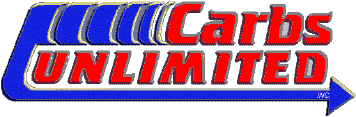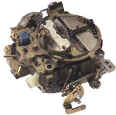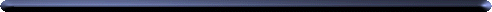General Ford Part Number decoding
For general reference onlyExample: # D1AF-DAB 1B 23

First Line
D
1AF-DA

Decade produced
B = 1950's
C = 1960's
D = 1970's
E = 1980's

D1AF-DA

Year produced
1 = 1971
2 = 1972
3 = 1973
ETC.
D1AF-DA Model , These do not always apply
A - Full size Ford / LTD , Etc.
D - Falcon , Maverick
G - Comet
L,V - Lincoln
M - Mercury
O - Mid size Ford / Fairlane , Torino
P - Replacement Part
S - Thunderbird
T - Truck
U - Bronco
Z - Mustang
D1AF-DA  - ?? If any one knows E-Mail Me
D1AF-DA  - ?? If any one knows E-Mail Me
B 1B 23 Design changes
Second Line
B
1B 23
4 Letter Date Code
Year Code
1 = 1971
2 = 1972
3 = 1973
ETC.
B 1B 23 Month of Date Code
 A = JanB = FebC = MarD = AprilE = MayF = June G = JulyH = AugJ = SeptK = OctL = NovM = Dec
B 1B 23 Day of Month
23rd day of the month
Example
1BF
3 Letter Date Code
Year Code
1 = 1971
2 = 1972
3 = 1973
ETC.
1BF Month of Date Code
 A = JanB = FebC = MarD = AprilE = MayF = June G = JulyH = AugJ = SeptK = OctL = NovM = Dec
1BF Week of Month
1 or A = 1st week
2 or B = 2st week
3 or C = 3st week
3 or D = 4st week
5 or E = 5st week